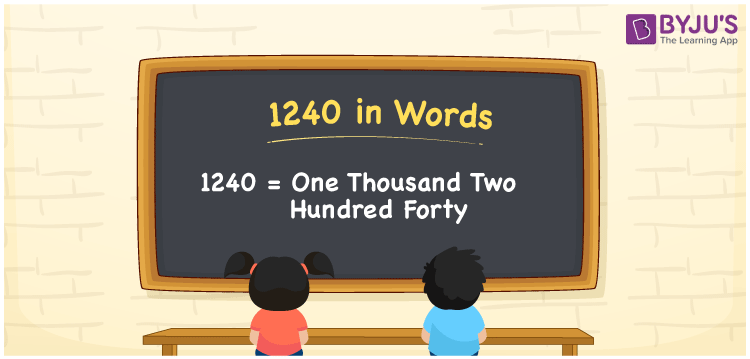# 1240 in words

1240 in words is written as One Thousand Two Hundred and Forty. 1240 represents the count or value. The article on Counting Numbers can give you an idea about counting. The number 1240 is used in expressions that relate to money, distance, length, year and others. Let us consider an example for 1240. “The cost of 3 kgs of Apples is One Thousand Two Hundred and Forty rupees.” Another example is, “The people gathered at a marriage were One Thousand Two Hundred and Forty.”

 1240 in words One Thousand Two Hundred and Forty One Thousand Two Hundred and Forty in Numerical Form 1240

## 1240 in English Words## How to Write 1240 in Words?

We can convert 1240 to words using a place value chart. The number 1240 has 4 digits, so let’s make a chart that shows the place value up to 4 digits.

 Thousands Hundreds Tens Ones 1 2 4 0

Thus, we can write the expanded form as:

1 × Thousand + 2 × Hundred + 4 × Ten + 0 × One

= 1 × 1000 + 2 × 100 + 4 × 10 + 0 × 1

= 1240

= One Thousand Two Hundred and Forty.

1240 is the natural number that is succeeded by 1239 and preceded by 1241.

1240 in words – One Thousand Two Hundred and Forty.

Is 1240 an odd number? – No.

Is 1240 an even number? – Yes.

Is 1240 a perfect square number? – No.

Is 1240 a perfect cube number? – No.

Is 1240 a prime number? – No.

Is 1240 a composite number? – Yes.

## Solved Example

Question:

Write the number 1240 in expanded form

Solution:

1240 = 1 × Thousands + 2 × Hundreds + 4 × Tens + 0 × Ones

= 1 × 1000 + 2 × 100 + 4 × 10 + 0 × 1

= 1000 + 200 + 40 + 0

= 1000 + 200 + 40

## Frequently Asked Questions on 1240 in words

Q1

### How to write 1240 in words?

1240 in words is written as One Thousand Two Hundred and Forty.
Q2

### State if True or False. 1240 is divisible by 2?

True. 1240 is divisible by 2.
Q3

### Is 1240 divisible by 10?

Yes. 1240 is divisible by 10.
Test your Knowledge on 1240 in Words